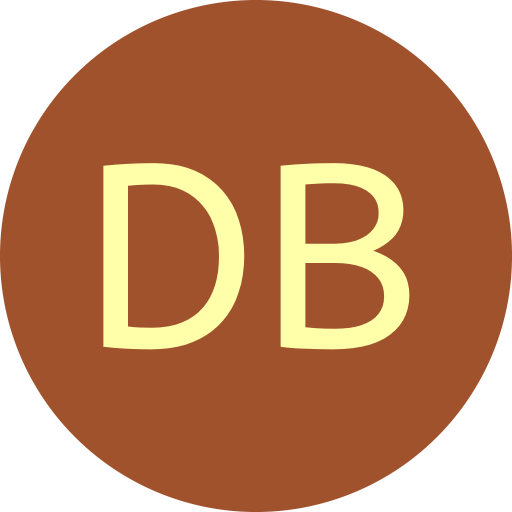#De Bruijn Sequence and Universal Cycle Constructions

Decoding the Granddaddy Output
 Algorithm Rank Unrank Order $n$ Symbols $k$ 2 3 4 5 6 7 8 9 String over $\{0,1,\ldots, k{-}1\}$
Decoding (ranking/unranking) the Granddaddy DB sequence

Given a DB sequence $\mathcal{D}$ of order $n$, the rank of a string $\alpha$ (of length $n$) is the starting index where $\alpha$ appears in $\mathcal{D}$. If $\alpha$ is a prefix of $\mathcal{D}$ then it has rank 1. If $\alpha$ starts at the last symbol of $\mathcal{D}$ and wraps around then it has rank $k^n.$ Unranking is the process of determining the string $\alpha$ in $\mathcal{D}$ starting at index $r$ (the rank of $\alpha$).

Example

Consider the Granddaddy DB sequence for $n=6$ and $k=2$.

0000001000011000101000111001001011001101001111010101110110111111

The string $\alpha = 010101$ has rank 47.

The only DB sequence construction presented in this project that has an efficient (polynomial-time) decoding algorithm is the Granddaddy [KRR16,SW17]. The ranking requires $O(n^2)$-time and the unranking requires $O(n^3 \log k)$-time (unit-cost RAM model) in the provided implementation based on [SW17]. The algorithms are based on efficient ranking algorithms for necklaces and Lyndon words. The only other known efficient decoding algorithms are for inter-leaved or inductive constructions [MEP96,Tul01] (coming soon).